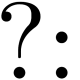Doug Smith - 3 years ago 91
C Question

# Why is my program apparently not reading all the input numbers, and how do I detect input that is not numerical?

For an assignment I have to code a program to do with hash tables. I've done that, and it seems to work well, only it's not producing the expected output if I input a variable at a specific point, and if I change it, it works (leading me to believe that it has to do with the order in which the numbers are read).

It's reading in as many numbers as the user inputs at the beginning, and then it hashes them and adds them to a hash table. It then figures out the amount of occurrences of that number, and if it's greater than the threshold the user enters (say user inputs 4 as the threshold, and there's 5 occurrences of 333) it will print out that number.

In my program, it should print 333 out, as to my count, it appears 8 times and I have the threshold set to 4. But it doesn't. If I change the output around so it appears within the first half of the input, it works. This makes me think it's only reading the numbers that are before LEN / 2 in the array (LEN is a value we were given to set as a macro).

Here's the code:

``````#include <stdio.h>

#define LEN 31

int main(int argc, char *argv[]) {
int keys[LEN];
int values[LEN];
int numAmount, threshold, i, j, num, index, counter, count, n, advance = 1;

// Set all indices of the arrays keys and values to 0
for (i = 0; i < LEN; i++) {
keys[i] = 0;
values[i] = 0;
}

scanf("%d %d", &numAmount, &threshold);
if (numAmount <= 0 || threshold <= 0) {
printf("\nLength of array and/or threshold is not a positive integer.");
}

// Main loop for adding numbers to the hash table
for (i = 0; i < numAmount && advance == 1; i++) {
counter = 0;
for (j = 0; j < LEN; j++) {
if (keys[j] != 0) {
counter++;
}
}
if (counter > LEN / 2) { // Load factor is greater than 1/2
printf("Load factor causes program to exit.");
break;
}

scanf("%d", &num);

// Illegal character detection
//      if (!num > 0) {
//          printf("Illegal character entry. Must be an integer from 0-9.");
//          break;
//      }

index = num % LEN;

if (keys[index] == 0) { // empty
keys[index] = num;
values[index] = 1;
}
else if (keys[index] == num) {
values[index]++;
}
else if (keys[index] != 0 && keys[index] != num) { // Collision
count = 0, n = 1;

while (keys[index] != 0 || keys[index] != num) { // Collision solver
if (count > LEN) {
break;
}
index = (index + n) % LEN;
n++;
count++;
}
}
}

// Only prints the results out if program went through with creating the hash table
for (i = 0; i < LEN; i++) {
if (values[i] >= threshold) {
printf("%d ", keys[i]);
values[i] = 0;
}
}
}
printf("\n");
}
``````

If I copy and paste this as input:

``````40 4
105 50 3 55 100
532 550 100 55 100
550 55 100 240 42
100 99 105 333 120
333 333 100 315 333
120 333 240 550 333
302 100 333 240 333
42 55 42 55 3
``````

It returns
`100 55`
, which is only 2/3 of the desired output; it's missing 333.

But if I put 333 near the beginning of the input, such as:

``````40 4
105 333 3 55 100
53 550 100 55 100
550 55 100 240 42
100 99 105 333 120
333 333 100 315 333
120 333 240 550 50
302 100 333 240 333
42 55 42 55 3
``````

It works as intended, and outputs
`100 333 55`
.

I've mulled over the code for awhile now, and I can't seem to figure out why this placement of the number is so crucial to the program working properly.

Also, quick secondary question, one of the things we're asked to do is make sure there is no illegal character input, basically anything non-numeric. How do I detect this? I know I could use an if statement (I have the structure of one commented out in the code above), but I'm not sure what the condition for the if statement should be. I know Regex, but Regex with C is confusing me greatly.jwodder
• You never do anything with `index` and `num` and whatnot after the collision solver loop, so the colliding value is simply discarded.
• Having the collision solver loop while `keys[index] != 0 || keys[index] != num` will cause it to reject any index that already corresponds to `num`, as such an index will already have a nonzero value in `keys`; change the `||` to a `&&`.
• Doing `index = (index + n) % LEN` causes `index` to continue accruing offsets, which (for `LEN` = 31, at least) leads to some indices being checked more than once and others not being checked at all. Do `index = (index + 1) % LEN` instead and dispense with `n` entirely.
As for detecting invalid input, simply test whether `scanf` returns 0 (indicating the next portion of the input failed to match `"%d"`) or not.